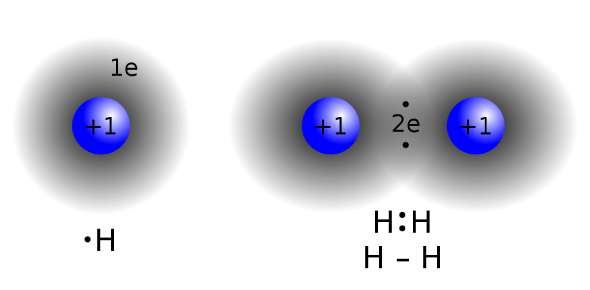# Covalent Bond Test! Trivia Quiz

8 Questions | Total Attempts: 509SettingsCovalent bonds are the chemical bonds that enable atoms to share electrons. There are different types of covalent bonds, depending on the number of electrons being shared. An example of a covalent body is the one where hydrogen and oxygen atoms bond. Do you know how to describe covalent bonds correctly? This quiz will help you refresh your memory on these types of bonds.

• 1.
What is a covalent bond?
• A.

The transfer of electrons from one atom to another atom

• B.

The sharing of electrons equally between atoms of non-metal in order to attain the (stable) electronic confirguration of a noble gas.

• 2.
Which of these is covalent bonding? i) σ-bonding ii) π-bonding iii) agostic interactions iv) three-center two-electron bonds
• A.

I) and iii)

• B.

I) only

• C.

Ii), iii) and iv)

• D.

All of the above

• 3.
What type of bond is present in this structural formula?X = X
• A.

Single Bond

• B.

Double Bond

• C.

Triple Bond

• 4.
What are the ions present in this dot and cross diagram?
• A.

Helium and Fluorine ions

• B.

Hydrogen and Sodium ions

• C.

Hydrogen and Flourine ions

• 5.
What is missing in this dot and cross diagram?
• A.

There should be 4 lone pairs of electrons added for the Oxygen ion.

• B.

Oxygen should share 4 electrons with 4 Hydrogen Ions.

• C.

There should be 2 lone pairs of electrons added for Hydrogen ions.

• 6.
What are the strongest type of covalent chemical bond?
• A.

Pi bond

• B.

Sigma Bond

• C.

Polar Bond

• D.

Non-Polar Bond

• 7.
Which pair of ions will form a double bond?
• A.

Hydrogen ions

• B.

Oxygen ions

• C.

Nitrogen ions

• 8.
What is the chemical formula for this dot-and-cross covalent bond diagram?
• A.

CaOH

• B.

2CaOH

• C.

Ca(OH)2

Related TopicsBack to top